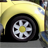# QlikView App Dev

Discussion Board for collaboration related to QlikView App Development.

Announcements
Join this live chat April 6, 10AM EST - QlikView to Qlik Sense REGISTER
cancel
Showing results for
Search instead for
Did you mean:Contributor II

## Comparing two Columns in a the same Chart

I have a chart with two columns of data both using the same Fieldname but existing in different states.

The expressions are:

=Sum({[Chart 1]} Fieldname)

=Sum({[Chart 2]} Fieldname)

I am trying to write a expression under the background color box that would compare the number in both columns and highlight them in yellow if the are different.  What I have written is below.  The expression is OK but it is not showing up as yellow where it is different.  Any idea on what is wrong in the expression?

=If(Aggr((Sum({[Chart 1]} [Addressable Spend])) <> (Sum({[Chart 2]} [Addressable Spend])), yellow())

1 Solution

Accepted SolutionsMVP

Why not just this:

If(Sum({[Chart 1]} Fieldname) <> Sum({[Chart 2]} Fieldname), Yellow())

4 RepliesMVP

Why not just this:

If(Sum({[Chart 1]} Fieldname) <> Sum({[Chart 2]} Fieldname), Yellow())Specialist

Probably

if(Coumn(1)<>Column(2),Yellow())Contributor II
Author

That worked perfectly.  Thanks for your help!MVP

No problem at all... Happy to help